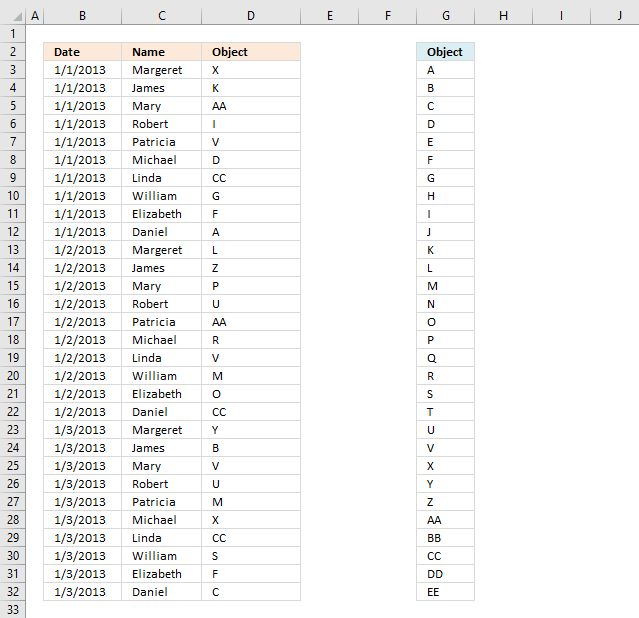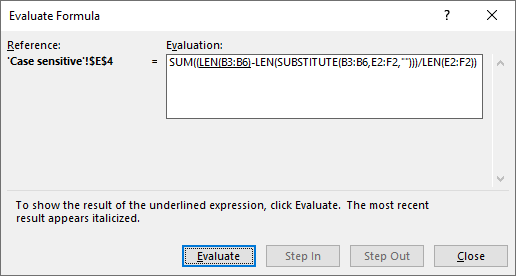Author: Oscar Cronquist Article last updated on February 05, 2020This article demonstrates a formula that distributes given text strings randomly across records in any given day meaning they may be repeated the next day.

The image above shows dates in column B, names in column C and a formula in column D that randomly extracts cell values from column G.

It will make sure that there are no duplicate text strings in any given day.

I've 30 objects and 10 people, and I need to assign each person randomly (unique) selected objects as a daily activity. Would you please suggest to me how I can do it using Excel formulae?

The objects are unique within that day. It means that a person can get the same object the next day if unlucky. If this is not what you are looking for, let me know.

The array formula in cell D3:D32 returns a random unique value every time you press F9. The objects are in column F.  The table does not need to be sorted by date.

Array formula in cell D3:

=INDEX(\$G\$3:\$G\$32,LARGE((COUNTIFS(\$D\$2:D2,\$G\$3:\$G\$32,\$B\$2:B2,B3)=0)*MATCH(ROW(\$G\$3:\$G\$32),ROW(\$G\$3:\$G\$32)),RANDBETWEEN(1,31-COUNTIF(\$B\$2:B3,B3))))

How to enter an array formula

1. Select cell D3
2. Copy above array formula.
3. Paste to cell C3.
4. Press and hold CTRL + SHIFT simultaneously.
5. Press ENTER once.
6. Release all keys.

The array formula begins and ends with curly brackets, like this: {=array_formula}. These characters appears automatically and indicate to the user that this is an array formula. Don't enter them yourself.

Note, the array formula returns new text strings randomly each time the worksheet is calculated.

Excel 365 subscribers have now access to dynamic arrays meaning they don't need to enter the formula as an array formula. Simply press Enter.

This formula returns a single value per cell and will not automatically spill values to cells below.

### Explaining array formula in cell D3I recommend that you use the "Evaluate Formula" tool located on the ribbon tab "Formulas" to examine any cell formula. This way you can see the all the calculation steps made and also more easily troubleshoot the formula.

1. Select cell D3.
2. Go to tab "Formulas" on the ribbon.
3. Press with left mouse button on the "Evaluate Formula" button and a dialog box appears, see image above.
4. Press with mouse on "Evaluate" button located on the dialog box to move to the next calculation step.
5. Continue with step 3 until you have seen all calculation steps or press with left mouse button on the "Close" button to dismiss the dialog box.

#### Step 1 - Identify unique text strings on a given day

The COUNTIFS function counts how many rows meet given criteria. In this case the COUNTIF function returns an array of values, each value in the array corresponds to the position in the unique text string list which is located in \$G\$3:\$G\$32.

COUNTIFS(criteria_range1criteria1, [criteria_range2criteria2]…)

COUNTIFS(\$D\$2:D2,\$G\$3:\$G\$32,\$B\$2:B2,B3)=0

becomes

{0; 0; 0; 0; 0; 0; 0; 0; 0; 0; 0; 0; 0; 0; 0; 0; 0; 0; 0; 0; 0; 0; 0; 0; 0; 0; 0; 0; 0; 0}=0

and returns

{TRUE; TRUE; TRUE; TRUE; TRUE; TRUE; TRUE; TRUE; TRUE; TRUE; TRUE; TRUE; TRUE; TRUE; TRUE; TRUE; TRUE; TRUE; TRUE; TRUE; TRUE; TRUE; TRUE; TRUE; TRUE; TRUE; TRUE; TRUE; TRUE; TRUE}

This means that all text strings can be randomly selected. If there had been previous object on the same day they would have been ruled out meaning their position in the array would be 1 or higher.

#### Step 2 - Calculate row numbers

The ROW function calculates the row number from each cell in cell range \$G\$3:\$G\$32 and returns an array of row numbers.

MATCH(ROW(\$G\$3:\$G\$32),ROW(\$G\$3:\$G\$32))

becomes

MATCH({3; 4; 5; 6; 7; 8; 9; 10; 11; 12; 13; 14; 15; 16; 17; 18; 19; 20; 21; 22; 23; 24; 25; 26; 27; 28; 29; 30; 31; 32},{3; 4; 5; 6; 7; 8; 9; 10; 11; 12; 13; 14; 15; 16; 17; 18; 19; 20; 21; 22; 23; 24; 25; 26; 27; 28; 29; 30; 31; 32})

The MATCH function calculates the relative position of each value in the array against the same array. This step makes sure that the array starts with 1 and increments with 1 up to the total number of values in cell range \$G\$3:\$G\$32.

MATCH({3; 4; 5; 6; 7; 8; 9; 10; 11; 12; 13; 14; 15; 16; 17; 18; 19; 20; 21; 22; 23; 24; 25; 26; 27; 28; 29; 30; 31; 32},{3; 4; 5; 6; 7; 8; 9; 10; 11; 12; 13; 14; 15; 16; 17; 18; 19; 20; 21; 22; 23; 24; 25; 26; 27; 28; 29; 30; 31; 32})

returns

{1; 2; 3; 4; 5; 6; 7; 8; 9; 10; 11; 12; 13; 14; 15; 16; 17; 18; 19; 20; 21; 22; 23; 24; 25; 26; 27; 28; 29; 30}

Now we need to multiply the arrays to get the corresponding row number. If the first array contains a zero the result will also be a 0 (zero) for that particular value in the array.

(COUNTIFS(\$D\$2:D2,\$G\$3:\$G\$32,\$B\$2:B2,B3)=0)*MATCH(ROW(\$G\$3:\$G\$32),ROW(\$G\$3:\$G\$32))

becomes

{TRUE; TRUE; TRUE; TRUE; TRUE; TRUE; TRUE; TRUE; TRUE; TRUE; TRUE; TRUE; TRUE; TRUE; TRUE; TRUE; TRUE; TRUE; TRUE; TRUE; TRUE; TRUE; TRUE; TRUE; TRUE; TRUE; TRUE; TRUE; TRUE; TRUE}*{1; 2; 3; 4; 5; 6; 7; 8; 9; 10; 11; 12; 13; 14; 15; 16; 17; 18; 19; 20; 21; 22; 23; 24; 25; 26; 27; 28; 29; 30}

and returns

{1; 2; 3; 4; 5; 6; 7; 8; 9; 10; 11; 12; 13; 14; 15; 16; 17; 18; 19; 20; 21; 22; 23; 24; 25; 26; 27; 28; 29; 30}

This array tells us that the formula in cell D3 can pick any of the text strings in column G which makes sense. None of the values have been displayed in cells above cell D3 for that particular date.

#### Step 3 - Return a random number

In this step, the COUNTIF function counts how many times the corresponding date for cell D3 has occurred in previous cells above.

There are 30 cells in column G that contains text strings, we can't return a random value from column G if we don't keep track of how many values we have displayed in cells above for that particular date.

The first argument in the COUNTIF function contains a cell reference that expands when you copy the cell and paste to cells below as far as needed.

RANDBETWEEN(1,31-COUNTIF(\$B\$2:B3,B3))

becomes

RANDBETWEEN(1, 31-1)

becomes

RANDBETWEEN(1, 30)

The RANDBETWEEN function returns a random value between the numbers you specify.

RANDBETWEEN(1, 30)

returns a random value between 1 and 30.

#### Step 4 - Return the k-th largest number

The LARGE function returns the k-th largest number from an array or cell range.

LARGE(array, k)

LARGE((COUNTIFS(\$D\$2:D2,\$G\$3:\$G\$32,\$B\$2:B2,B3)=0)*MATCH(ROW(\$G\$3:\$G\$32),ROW(\$G\$3:\$G\$32)),RANDBETWEEN(1,31-COUNTIF(\$B\$2:B3,B3)))

becomes

LARGE({1; 2; 3; 4; 5; 6; 7; 8; 9; 10; 11; 12; 13; 14; 15; 16; 17; 18; 19; 20; 21; 22; 23; 24; 25; 26; 27; 28; 29; 30}, RANDBETWEEN(1, 30))

and returns a random row number between 1 and 30.

#### Step 5 - Return a value

The INDEX function returns a value from cell range \$G\$3:\$G\$32 based on the random row number we calculated in the previous step.

INDEX(\$G\$3:\$G\$32,LARGE((COUNTIFS(\$D\$2:D2,\$G\$3:\$G\$32,\$B\$2:B2,B3)=0)*MATCH(ROW(\$G\$3:\$G\$32),ROW(\$G\$3:\$G\$32)),RANDBETWEEN(1,31-COUNTIF(\$B\$2:B3,B3))))

becomes

=INDEX(\$G\$3:\$G\$32, random_row_number)

becomes

INDEX({"A"; "B"; "C"; "D"; "E"; "F"; "G"; "H"; "I"; "J"; "K"; "L"; "M"; "N"; "O"; "P"; "Q"; "R"; "S"; "T"; "U"; "V"; "X"; "Y"; "Z"; "AA"; "BB"; "CC"; "DD"; "EE"}, random_row_number)

and returns a random text string.